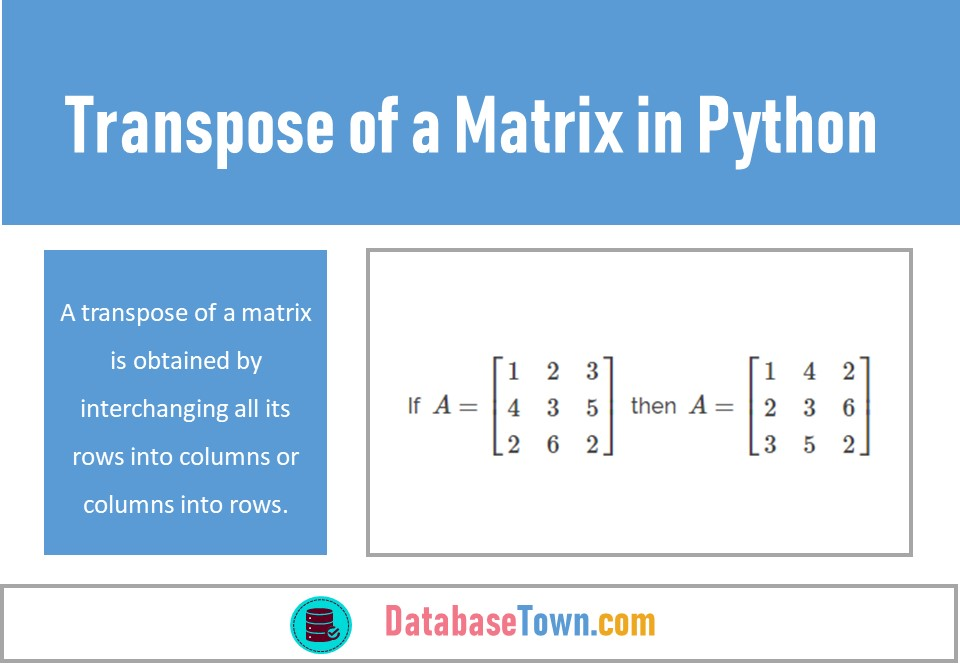# Transpose of a Matrix in Python

A transpose of a matrix is obtained by interchanging all its rows into columns or columns into rows. It is denoted by $$\displaystyle {{A}^{t}}$$ or $$\displaystyle {{A}^{‘}}$$. For example,

If  $$\displaystyle A=\left[ {\begin{array}{*{20}{c}} 1 & 2 & 3 \\ 4 & 3 & 5 \\ 2 & 6 & 2 \end{array}} \right]\,$$ then  $$\displaystyle A=\left[ {\begin{array}{*{20}{c}} 1 & 4 & 2 \\ 2 & 3 & 6 \\ 3 & 5 & 2 \end{array}} \right]$$

In transpose of a matrix, the values of matrix are not changing, only their positions are changing. When we take the transpose of a same vector two times, we again obtain the initial vector. Further, A m x n matrix transposed will be a n x m matrix as all the rows of a matrix turn into columns and vice versa.

Let’s start a practical example of taking a transpose of a matrix in python…

First, we import the relevant libraries in Jupyter Notebook. We use NumPy, a library for the python programming that allows us to work with multidimensional arrays and matrices along with a large collection of high-level mathematical functions to operate on these arrays as shown below,

## Transpose of a Matrix

Let’s see more examples of transpose of a matrix …

In above examples, you can see that, only rows of two matrices B and C are changed into columns and columns into rows.

Let’s see an example of taking transpose of a scalar and a vector.

It transpires from above examples that when we take a transpose of a scalar or vector, we obtain the same result because in python one dimensional array do not get transposed.

However, if we intend to get the transpose of a vector then we will reshape it into 4 x 1 matrix or 2-dimensional array, let’s do it practically,